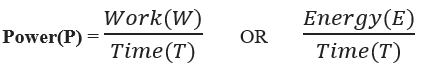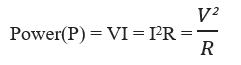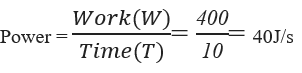# Power Formula

## Power Formulas - Electrical Power Formula Derivations & Examples

Power is defined as work done in unit time or energy used in unit time.
So mathematically,Where T is the time taken to do the work or time taken for which the energy is used.
SI unit of power is J/s.
In an electrical circuitWhere V = Potential across a resistor
I = Current Passing through the resistor
R = Resistance
SI unit of Power used is Watt.

Example: How much Power needs to be used to lift a mass of 5Kg to a height of 8m in 10s.(Take g = 10m/s2)
Ans:
Work needs to be done in lifting an object to a height = potential energy at that height
Therefore, Work(W) = mgh = 5 x 8 x 10 = 400J.Question:
A motor with a power of 4W is used continuously to move an object of mass 2Kg for 16s. Find the velocity with which the object will move after 16s.